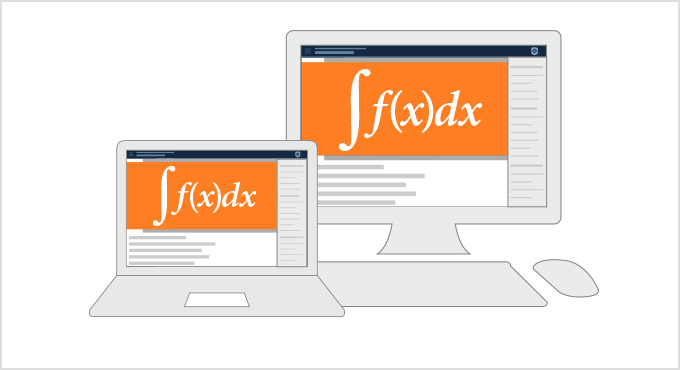#Wolfram U

FULL INTERACTIVE COURSE

# Introduction to Calculus

Level: Beginner### Summary

A comprehensive introduction to fundamental concepts in calculus, including video lessons and interactive notebooks. Follow along with the examples in the Wolfram Cloud and use the material to prepare for the AP Calculus AB exam. The course starts with functions and limits, followed by differential calculus and its applications, and then moves on to integral calculus and its applications. Problem sessions, exercises, quizzes and a sample exam are provided for self-paced assessment. Earn a certificate by watching all lesson and problem session videos and completing the quizzes with a passing grade.

Prerequisites for calculus are Algebra I (elementary algebra), Algebra II (intermediate algebra), elementary geometry and trigonometry or precalculus. Recommended best practice for completing this interactive course is to start with Lesson 1 and progress through the video lessons, working through each problem session and taking each quiz in the order it appears in the table of contents.

### You'll Learn To

Work with functions and limits

Solve problems using derivatives

Use indefinite and definite integrals

Relate concepts of differentiating and integrating functions

Solve separable differential equations

Prepare for AP CalculusAsk questions or start a discussion about related topics on Wolfram Community.